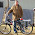## Sunday

### Monitoring voltage of a dc battery supply

Since we are involved in off grid solar power systems, we have a need to monitor battery voltage. The Arduino can do this easily with a simple voltage divider. With some simple mods, we can control loads, generators, or notifications based on battery voltage.

To read a maximum of 20vdc, R1 should be 3k ohm, R2 should be 1k ohm, and the code would be as follows:

/*
DisplayMoreThan5V sketch
prints the voltage on analog pin to the serial port
Do not connect more than 5 volts directly to an Arduino pin.
*/

const int referenceVolts = 5; // the default reference on a 5-volt board
//const float referenceVolts = 3.3; // use this for a 3.3-volt board

const int R1 = 3000; // value for a maximum voltage of 20 volts
const int R2 = 1000;
// determine by voltage divider resistors, see text
const int resistorFactor = 255 / (R2/(R1 + R2));
const int batteryPin = 0; // +V from battery is connected to analog pin 0

void setup()
{
Serial.begin(9600);
}

void loop()
{
int val = analogRead(batteryPin); // read the value from the sensor
float volts = (val / resistorFactor) * referenceVolts ; // calculate the ratio
Serial.println(volts); // print the value in volts
}

This example was based on info in Arduino Cookbook (Oreilly Cookbooks)1.I want to ask you something stupid but do i have to use regular resistors (small ones) or some special bigger ones? Cause i want to measure voltage from 2 parallel 12v batteries from a truck.

2.The wattage of the resistor is depended upon the power running through it. Since all we are doing is "watching" the voltage, not powering a load" the power consumption is very low, so 1/4 or 1/2 watt resistors will work fine.

3.I think there is an error in the calculation of resistorFactor:

According to the example in the book you should divide the resolution of analogRead(), i.e. 1023 by R2(R1+R2) to get 255.

At least I think so..

4.Does it make a difference if the Arduino is running of the measured source, ie. could you wire the +V to the VIN pin?

1.You could if +v is 5v. Otherwise if it's 7-12v, use the power port.

2.Well the whole idea is that I don't know what +V is... What is the power port? On an Uno for example I have 3.3v, 5v, and VIN. VIN would be coming from a battery or whatever *into* the Arduino, correct?

For whatever reason it doesn't seem right to be measuring the voltage in a system that's powering itself. Thanks.

5.The barrel connector is the power port on the arduino. It can take 7-12v or so. Vin can only be 5v, and the voltage divider +V is higher than that (mine is about 16v). Why do you have a problem with monitoring the same power source? We do it all the time.

6.I'm no expert diagram reader but it looks like VIN and the positive barrel jack post are connected, only separated by a diode (presumably to prevent back feeding the barrel?) http://circuitdiagram.net/wp-content/uploads/2011/06/arduino-uno-schematic.jpg

I assumed this concept would be easy originally, maybe even with software alone (I'm a software guy ;) but I found nothing and I recall reading that it wasn't possible. I should know better than to listen to the Internet.

Anyway, thanks for the post and comments.

7.The barrel connector feeds the voltage regulator. Apparently, so does Vin. You are correct in that regard.

8.Would this work to measure the voltage of a 3.7v Li-Ion battery? Will the resistors be the same?

9.You can measure a 3.7v battery, but the resistor values would be different. See http://arduinotronics.blogspot.com/2012/04/voltage-monitor.html

10.Hi! Is it possible to have two DC voltage sensors connected to the same Arudino board?

1.11.Hi! Wont the current be too high with the grounds on the Arudino board being connected to each other?

1.I'm not understanding your question. There is little current flow, due to the high impedance of the input. What grounds are you referring to? A 12k R1 and 5k R2 resistor will reduce power consumption.

12.I was having trouble with this, so to help others coming by this post, you should know that your Arduino and your voltage source have to share a common ground. This is one of those things I think people experienced in electronics would just know, but those of us coming at this from software don't.

Without the common ground, I was getting really erratic readings on my analog in. (Like 0, then 5.01 volts, then 0, then 2.3 volts, then 0, etc.) My Arduino was getting power from USB, my voltage divider was getting power and ground from my battery. So my Arduino analog in was getting the result of the voltage coming out of the voltage divider. But there was no connection between the grounds (so in the diagram above, the GND at the bottom right wasn't connected to the GND on my battery).

When I interpreted the diagram with the GND at the bottom right as sticking off a bit from that junction, like the +V, I tried tying my grounds together. The result: rock solid readings from my voltage divider, with a reading right where I would expect based on my resistors and my battery.

I suspect if I were powering the Arduino from my battery there wouldn't be an issue, since the Arduino's ground would *be* the battery's ground.

13.Yes, the grounds have to be common in all Arduino projects where you have separate power sources, unless isolation is a requirement, like with optoisolators.

14.Je veux savoir comment brancher les fils de la batterie sur l'arduino MKRZERO pour l'alimenter et commente on doit charger cette batterie???

1.mkr zero pinout are found at https://store.arduino.cc/usa/arduino-mkrzero